# Self Referential Formula, Plot your name!

Hello Guys;

I wrote a note before that about a website that where you can graph your name.

Today I'll present to you this formula :

$\frac{1}{2} < \left\lfloor \bmod\left(\left\lfloor\frac{y}{17}\right\rfloor2^{-17\lfloor x\rfloor - \bmod(\lfloor y \rfloor, 17)}, 2\right)\right\rfloor$

its name is Tupper's Self Referential Formula and the best part of this is that when we plot it in certain range the graph is the formula itself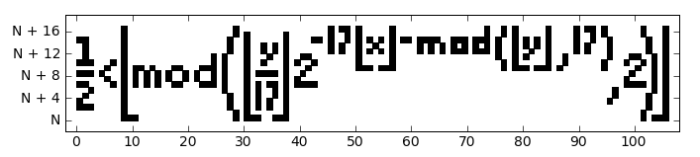Amazing isn't it and the number $N$ is equal to:

 1 N=4858450636189713423582095962494202044581400587983244549483093085061934704708809928450644769865524364849997247024915119110411605739177407856919754326571855442057210445735883681829823754139634338225199452191651284348332905131193199953502413758765239264874613394906870130562295813219481113685339535565290850023875092856892694555974281546386510730049106723058933586052544096664351265349363643957125565695936815184334857605266940161251266951421550539554519153785457525756590740540157929001765967965480064427829131488548259914721248506352686630476300 

That's not everything. The images produced by Tupper’s formula are black and white pictures 106 pixels wide by 17 pixels high. If you take a 106 × 17 grid and place a 1 in the squares you want to be black and a 0 in the squares you want to be white, rotating the image and reading the digits off left to right, working down the image, will give you a 1,802-digit binary number. If you convert that number into base-10, then multiply it by 17, you get the value $$N). like if we want to plot the name \(KAITO$$ we do this: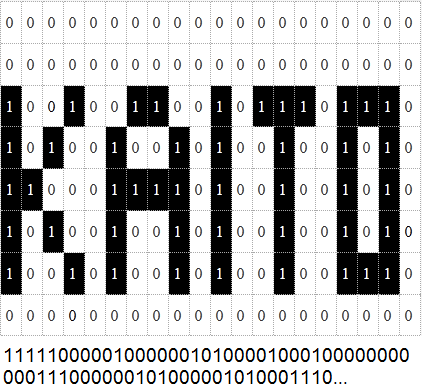and this Python code allow you to plot the formula for any integer $N$:

  1 2 3 4 5 6 7 8 9 10 11 12 13 14 15 16 17 18 19 20 21 22 23 24 25 26 27 28 29 30 31 32 33 34 35 36 37 38 39 40 41 42 43 44 45 46 47 48 #!/usr/bin/env python # -*- coding: utf-8 -*- """ Plot Tupper's self-referential formula """ #N = 960939379918958884971672962127852754715004339660129306651505519271702802395266424689642842174350718121267153782770623355993237280874144307891325963941337723487857735749823926629715517173716995165232890538221612403238855866184013235585136048828693337902491454229288667081096184496091705183454067827731551705405381627380967602565625016981482083418783163849115590225610003652351370343874461848378737238198224849863465033159410054974700593138339226497249461751545728366702369745461014655997933798537483143786841806593422227898388722980000748404719 N = 4858450636189713423582095962494202044581400587983244549483093085061934704708809928450644769865524364849997247024915119110411605739177407856919754326571855442057210445735883681829823754139634338225199452191651284348332905131193199953502413758765239264874613394906870130562295813219481113685339535565290850023875092856892694555974281546386510730049106723058933586052544096664351265349363643957125565695936815184334857605266940161251266951421550539554519153785457525756590740540157929001765967965480064427829131488548259914721248506352686630476300 H = 17 W = 1 import sys if __name__ == '__main__': if len(sys.argv)>1: H = int(sys.argv) def tupper(x,y): return 0.5 < ((y//H) // (2**(H*x + y%H))) % 2 print "x range: 0 < x <", W = int(raw_input()) print 'Got width: %d' % W print "y range: N < y < N+%d, where N = (type 0 for default)" % H, t = int(raw_input()) if t: N=t print import matplotlib.pyplot as plot plot.rc('patch', antialiased=False) print 'Plotting...' for x in xrange(W): print 'Column %d...' % x for yy in xrange(H): y = N + yy if tupper(x,y): plot.bar(left=x, bottom=yy, height=1, width=1, linewidth=0, color='black') print 'Done plotting, please wait...' plot.axis('scaled') #For large graphs, must change these values (smaller font size, wider-apart ticks) buf = 2 plot.xlim((-buf,W+buf)) plot.ylim((-buf,H+buf)) plot.rc('font', size=10) plot.xticks(range(0, W, 100)) yticks = range(0, H+1, 4) plot.yticks(yticks, ['N']+['N + %d'%i for i in yticks][1:]) plot.savefig('out.png') plot.savefig('out.svg') 

and Finally i calculate the $N$ of some names of brilliant's members those who amazed me with their Ideas Problems and Solutions, Hope they don't Mind

starting with brilliant@Calvin Lin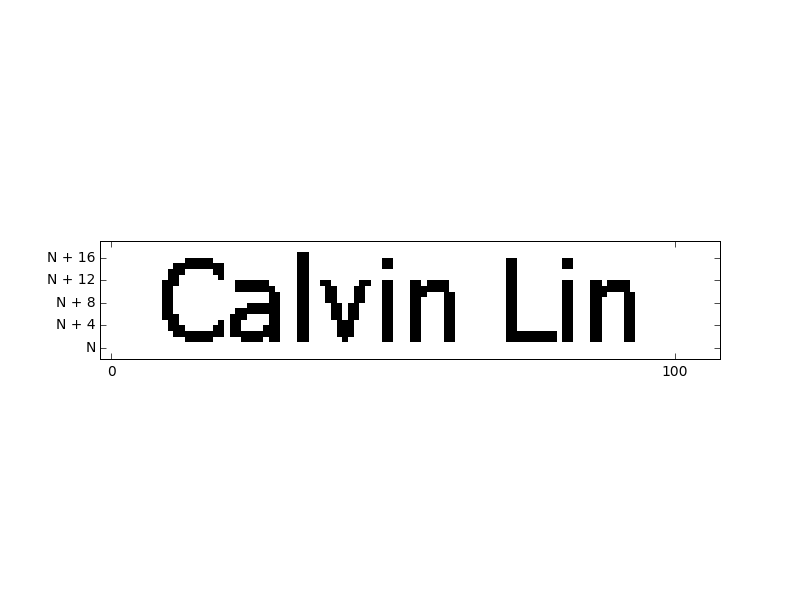@Pi Han Goh@Jake Lai@Chew-Seong Cheong@Nihar Mahajan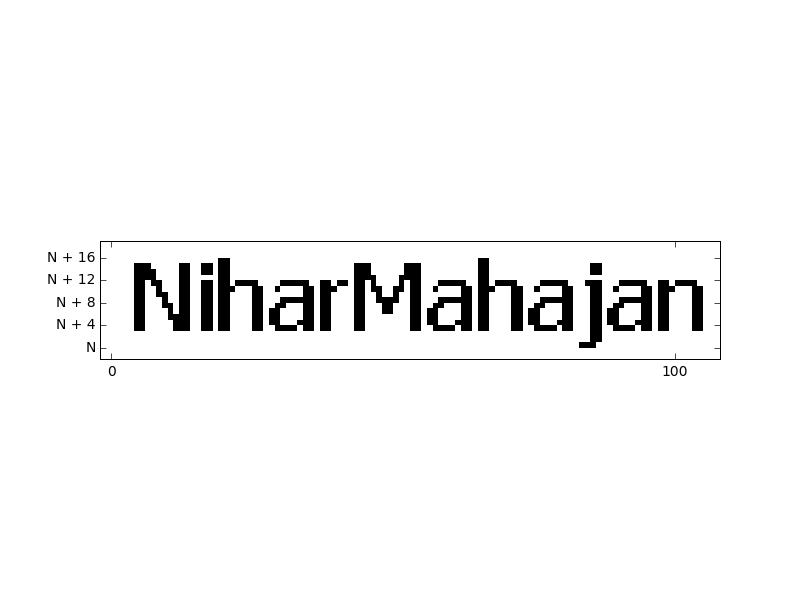@Satyajit Mohanty@Maggie Miller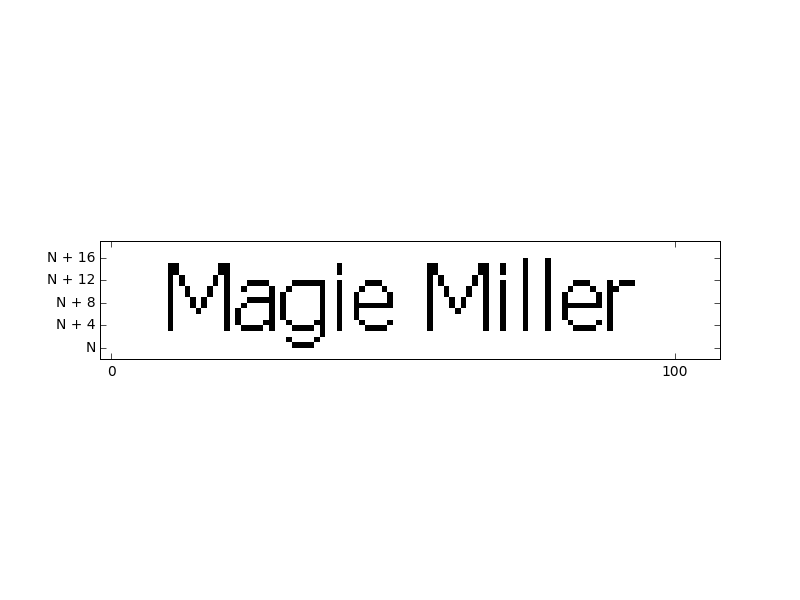For the numbers $N$ for every name you can find them Here

feel free to comment anything any suggest... See you another time ;)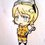Note by Abdeslem Smahi
5 years, 10 months ago

This discussion board is a place to discuss our Daily Challenges and the math and science related to those challenges. Explanations are more than just a solution — they should explain the steps and thinking strategies that you used to obtain the solution. Comments should further the discussion of math and science.

When posting on Brilliant:

• Use the emojis to react to an explanation, whether you're congratulating a job well done , or just really confused .
• Ask specific questions about the challenge or the steps in somebody's explanation. Well-posed questions can add a lot to the discussion, but posting "I don't understand!" doesn't help anyone.
• Try to contribute something new to the discussion, whether it is an extension, generalization or other idea related to the challenge.
• Stay on topic — we're all here to learn more about math and science, not to hear about your favorite get-rich-quick scheme or current world events.

MarkdownAppears as
*italics* or _italics_ italics
**bold** or __bold__ bold
- bulleted- list
• bulleted
• list
1. numbered2. list
1. numbered
2. list
Note: you must add a full line of space before and after lists for them to show up correctly
paragraph 1paragraph 2

paragraph 1

paragraph 2

[example link](https://brilliant.org)example link
> This is a quote
This is a quote
    # I indented these lines
# 4 spaces, and now they show
# up as a code block.

print "hello world"
# I indented these lines
# 4 spaces, and now they show
# up as a code block.

print "hello world"
MathAppears as
Remember to wrap math in $$ ... $$ or $ ... $ to ensure proper formatting.
2 \times 3 $2 \times 3$
2^{34} $2^{34}$
a_{i-1} $a_{i-1}$
\frac{2}{3} $\frac{2}{3}$
\sqrt{2} $\sqrt{2}$
\sum_{i=1}^3 $\sum_{i=1}^3$
\sin \theta $\sin \theta$
\boxed{123} $\boxed{123}$

## Comments

Sort by:

Top Newest

I saw this on Numberphile

- 5 years, 10 months ago

Log in to reply

Yes they made a video about i think He was Matt Parker who made it

- 5 years, 10 months ago

Log in to reply

Yeah matt parker it was

- 5 years, 10 months ago

Log in to reply

Okay, my understanding is that a "self-referential formula" is like a mechanism that builds a replica of itself, i.e., it reproduces. If you have a name, and it's rasterized and converted to a number, how is that self-reproduction? Looking at this from a different direction, suppose I have a class of formulas or algorithms in which to generate a sequence of numbers. Further suppose that we have some quote, say, "I am computed, therefore I am". Is it possible to design a formula or algorithm where it is not obvious how that quote could have been "programmed into it", and yet, somewhere in the sequence of numbers, put in raster form, that quote pops out, thus, "I am computed, therefore I am"? Furthermore, how about if that quote is the actual formula being used to generate it? That was Tupper's feat, which is what makes it so interesting.

- 5 years, 10 months ago

Log in to reply

Dude , Amazing! SuperLike :)

- 5 years, 10 months ago

Log in to reply

Only coz he plotted your name too :P

Jk Great work! @Kaito Einstein

- 5 years, 10 months ago

Log in to reply

He plotted my name because I am his friend. Indeed the work is quite amazing and even if my name was not plotted , my opinion would remain unchanged.I hope this changes your view too. :)

- 5 years, 10 months ago

Log in to reply

@Nihar Mahajan Tum toh senti ho gye yaar :3

I was just joking man! Each member of the community is everyone's friend. :)

- 5 years, 10 months ago

Log in to reply

Nothing sentimental in that. Since you had got the wrong reason ,I just gave the right reason why I posted that comment.

- 5 years, 10 months ago

Log in to reply

Okay dude, Relax!

- 5 years, 10 months ago

Log in to reply

I am already relaxed (for some time) :)

- 5 years, 10 months ago

Log in to reply

Good to know. :)

- 5 years, 10 months ago

Log in to reply

Calvin Lin Chew-Seong Cheong Maggie Miller Jake Lai Pi Han Goh

- 5 years, 10 months ago

Log in to reply

Can you please do it in my name too ? In the comment box !

- 5 years, 10 months ago

Log in to reply

ok no problem but it will take some time, maybe after 4 hours :)

- 5 years, 10 months ago

Log in to reply

Thanks

- 5 years, 10 months ago

Log in to reply

Sorry i was busy with the wiki stuff Here it isand your number

 1 2 3 4 5 6 7 N=14913018598508217101155688768234267522813695994543056967689749189606269039948145 17947455467599239313784050786329341834120655005268376606772574316195944803363933 90603570161219336303076442802043801760927552257534594673414915222277281331802168 58256891645222468510251029398970333540489706205269797440078968914235597074299744 76404648259174702889225835326834679481407149474957297471699368451716522429625405 38915618418710658562274139127367528730029959519508336717050975409039817806424933 50295118443910333683764555677696 

- 5 years, 10 months ago

Log in to reply

Great Work , Kaito !

- 5 years, 10 months ago

Log in to reply

Thanks :)

- 5 years, 10 months ago

Log in to reply

Nice. I just found about the function (here's the graph - desmos).

Here's an interesting link - tupper formula

- 5 years, 2 months ago

Log in to reply

×

Problem Loading...

Note Loading...

Set Loading...